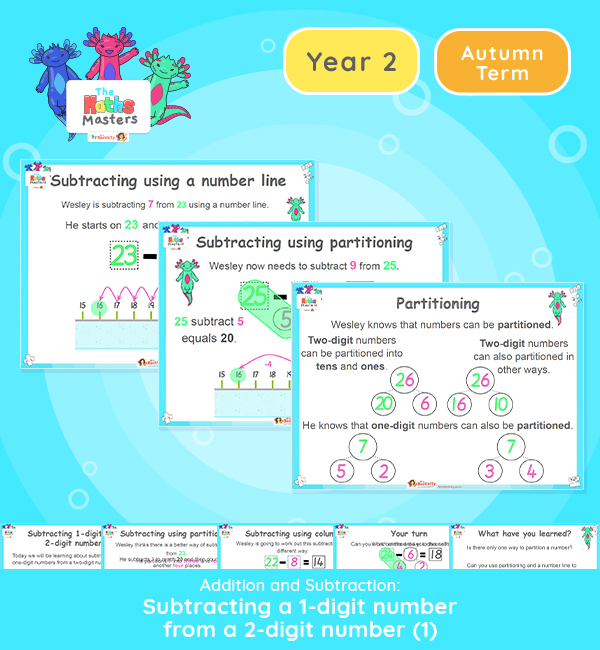# Year 2 | Subtracting a 1 Digit From a 2 Digit Number Part 1 Lesson Presentation## White Rose Year 2 Addition and Subtraction resources

Aligned with the White Rose Maths scheme of work, this Year 2 | Subtracting a 1 Digit From a 2 Digit Number Lesson Presentation is fully editable, and is designed for the Year 2 maths curriculum covering the following maths objectives for the autumn term:

This is the first lesson of two lessons subtracting a one-digit number from a two-digit number and crossing a tens boundary. It illustrates how to use both the number line and column method of subtraction.

White Rose Maths Small Steps: Subtract a 1-digit from a 2-digit number – crossing ten.

NC Links: Recall and use addition and subtraction facts to 20 fluently. Add and subtract numbers using concrete objects, pictorial representations and mentally.

White Rose Maths Small Steps: Subtract a 1-digit from a 2-digit number – crossing ten.

NC Links: Recall and use addition and subtraction facts to 20 fluently. Add and subtract numbers using concrete objects, pictorial representations and mentally, including a two-digit number and ones.

Solve problems with addition and subtraction: using concrete objects and pictorial methods

Year 1 Conceptual Prerequisites: Add and subtract within 10. Know that a multiple of 10 is made up of a number of tens.

2AS-3 Add and  subtract within 100 by applying related one-digit addition and subtraction facts: add and subtract only ones or only tens to/from a two-digit number

Future applications: Add and subtract using mental and formal written methods.

TAF Statements:     Working Towards: Add and subtract (one-digit numbers) explaining their method verbally, in pictures, or using apparatus. Recall at least four of the six number bonds for 10 and reason about associated facts.

Working At: Recall all the number bonds to and within 10, and use these to reason with a calculate bonds to and within 20, recognising associated additive relationships.

Greater depth – Using reasoning about numbers and relationships to solve more complex problems and explain their thinking.

Aligned with the order of teaching of the White Rose Maths scheme of work, use this to help your class get to grips with each mathematical concept. This lesson presentation also includes varied fluency activities, problem solving, and mathematical discussion questions too.

Explore our other  year 2 addition and subtraction resources.